Checkout JEE MAINS 2022 Question Paper Analysis : Checkout JEE MAINS 2022 Question Paper Analysis :

# Important Questions for Class 11 Chemistry Chapter 4 – Chemical Bonding and Molecular Structure.

Class 11 chemistry important questions with answers are provided here for Chapter 4 Chemical Bonding and Molecular Structure. These important questions are based on the CBSE board curriculum and correspond to the most recent Class 11 chemistry syllabus. By practising these Class 11 important questions, students will be able to quickly review all of the ideas covered in the chapter and prepare for the Class 11 Annual examinations as well as other entrance exams such as NEET and JEE.

Download Class 11 Chemistry Chapter 4 Chemical Bonding and Molecular Structure Important Questions with Answers PDF by clicking on the button below.

## Class 11 Chemical Bonding and Molecular Structure Important Questions with Answers

1. Explain the non linear shape of H2S and non planar shape of PCl3 using valence shell electron pair repulsion theory.

• The main atom in H2S is S, which has two lone pairs. These lone pairs cause repulsion and displace the H-S bond, resulting in a non-linear shape.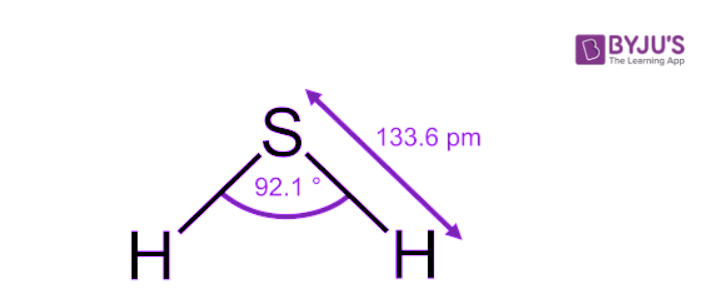• PCl3 has a trigonal planar structure. P has three single bonds and one lone pair (pair of unshared electrons). Each chlorine atom has a single 3p orbital that is completely occupied. The overlap of a phosphorus sp3 hybrid orbital with a singly occupied chlorine 3p orbital results in the formation of P–Cl bonds. Three lone pairs are held by each Cl atom.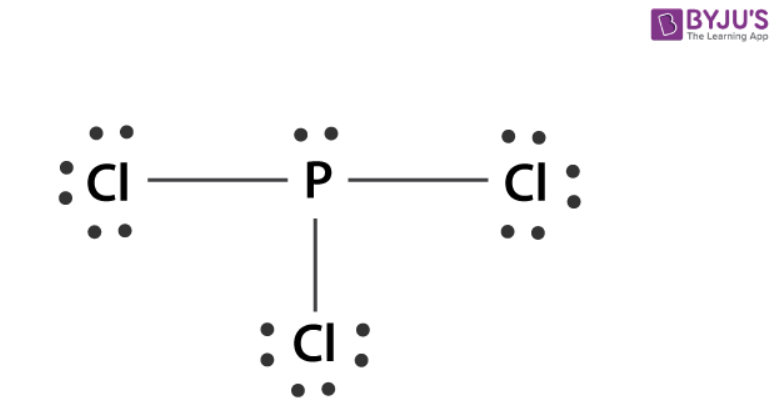2. Using molecular orbital theory, compare the bond energy and magnetic character of O2+ and O2 species.

The electronic configurations of O2+ and O2 species are as follows: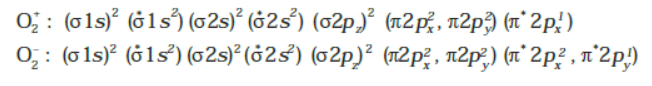In O2+ the bond order is (10 – 5)/2 = 2.5

In O2 the bond order is (10 – 7)/2 = 1.5

Bond dissociation energy is directly proportional to bond order, and unpaired electrons determine paramagnetic character. Both O2+ and O2 have high dissociation energy and are paramagnetic.

3. Explain the shape of BrF5.

In BrF5 the central atom Br is surrounded by five bonded pairs and one lone pair. This forms the shape of square pyramidal.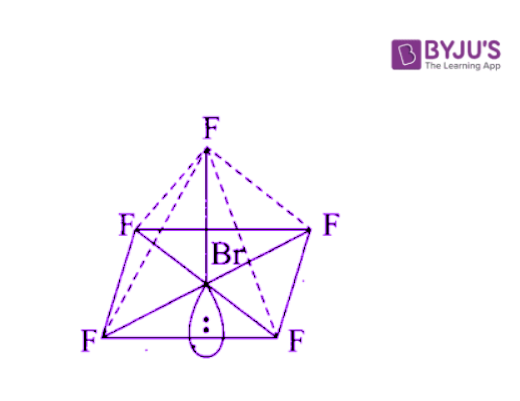4. Structures of molecules of two compounds are given below:

(a) Which of the two compounds will have intermolecular hydrogen bonding and which compound is expected to show intramolecular hydrogen bonding.

(b) The melting point of a compound depends on, among other things, the extent of hydrogen bonding. On this basis explain which of the above two compounds will show higher melting point.

(c) Solubility of compounds in water depends on power to form hydrogen bonds with water. Which of the above compounds will form a hydrogen bond with water easily and be more soluble in it.

(a) Since the NO2 and OH groups in compound (I) are close together, intramolecular hydrogen bonding will form (II). Compound (II) will show intermolecular hydrogen bonding.

(b) Since it forms intramolecular hydrogen bonds, compound (II) has a higher melting point. As a result, more and more molecules are linked together via hydrogen bond formation.

(c) Due to intramolecular hydrogen bonding, compound (I) cannot form hydrogen bonds with water and is thus less soluble in it, whereas compound (II) can form hydrogen bonds with water more easily and is thus more soluble in water.

5. Why does type of overlap given in the following figure not result in bond formation?

• In the first figure, the ++ overlap equals the +- overlap, so these cancel out and the net overlap is zero.
• Since the two orbitals are perpendicular to each other in the second figure, no overlap is possible.

6. Explain why PCl5 is trigonal bipyramidal whereas IF5 is square pyramidal.

P is surrounded by 5 bond pairs and no lone pairs in PCl5, whereas iodine atom is surrounded by 5 bond pairs and one lone pair in IF5, so the shape of PCl5 is trigonal bipyramidal and IF5 is square pyramidal

7. In both water and dimethyl ether (CH3–O–CH3), oxygen atom is central atom, and has the same hybridization, yet they have different bond angles. Which one has a greater bond angle? Give reason.

The bond angle of dimethyl ether will be greater. More repulsion will exist between bond pairs of CH3 groups attached in ether than between bond pairs of hydrogen atoms attached to oxygen in the water.

The carbon of CH3 in ether is attached to three hydrogen atoms via bonds, and the electron pair of these bonds contribute to the electronic charge density on the carbon atom. As a result, the repulsion between two CH3 groups will be greater than that between two hydrogen atoms.

8. Write Lewis structure of the following compounds and show a formal charge on each atom. HNO2, NO2, H2SO4

• The Lewis structure of HNO3 is-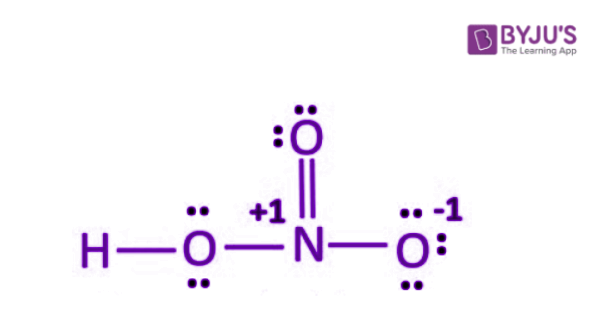Formal charge = Valence Electrons – Unbonded Electrons – ½ Bonded Electrons

Formal charge on O that has double bond = 6 – 4 –½ (4) = 0
Formal charge on O that is attached to H atom = 6 – 4 –½ (4) = 0
Formal charge on O that has single bond = 6 – 6 –½ (2) = – 1
Formal charge on H = 1 – 0 – ½ (2) = 0
Formal charge on N = 5 – 0 – ½ (8) = +1

• The Lewis structure of NO2 is-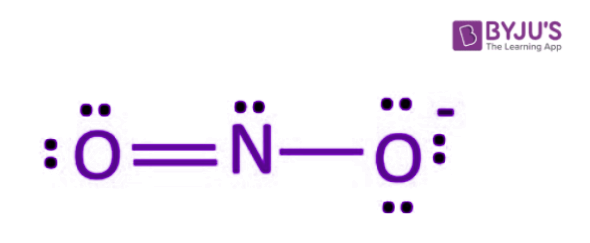Formal charge = Valence Electrons – Unbonded Electrons – ½ Bonded Electrons
Formal charge on O that has double bond = 6 – 4 –½ (4) = 0
Formal charge on O that has single bond = 6 – 6 –½ (2) = – 1
Formal charge on N = 5 – 2 – ½ (6) = 0

• The Lewis structure of H2SO4 is-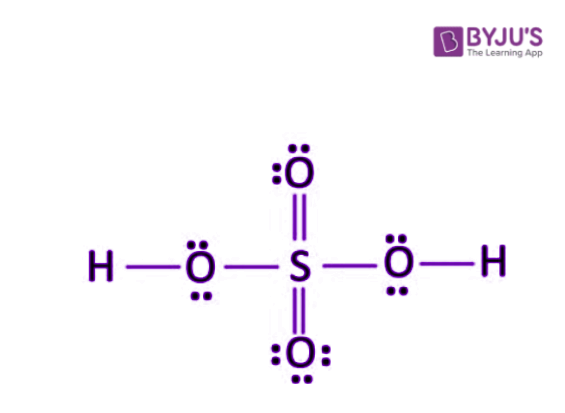Formal charge = Valence Electrons – Unbonded Electrons – ½ Bonded Electrons
Formal charge on O that has double bond = 6 – 4 –½ (4) = 0
Formal charge on O that has single bond = 6 – 4 –½ (4) = 0
Formal charge on H = 1 – 0 – ½ (2) = 0
Formal charge on S = 6 – 0 – ½ (12) = 0

9. The energy of σ2pz molecular orbital is greater than π2px and π2py molecular orbitals in nitrogen molecule. Write the complete sequence of energy levels in the increasing order of energy in the molecule. Compare the relative stability and the magnetic behaviour of the following species: N2, N2+ , N2 , N22+

1. N2 molecule has electronic configuration-
σ1s2, σ∗1s2, σ2s2, σ∗2s2, π2px2 = π2py2, σ2pz2
Here, Nb = 10, Na = 4
Hence, Bond Order = ½ (Nb – Na) = ½ (10 – 4) = 3
The presence of no unpaired electron indicates it to be diamagnetic.
2. N2+ molecule has electronic configuration-
σ1s2, σ∗1s2, σ2s2, σ∗2s2, π2px2 = π2py2, σ2pz1
Here, Nb = 9, Na = 4
Hence, Bond Order = ½ (Nb – Na) = ½ (9 – 4) = 2.5
The presence of 1 unpaired electron indicates it to be paramagnetic.
3. N2 molecule has electronic configuration-
σ1s2, σ∗1s2, σ2s2, σ∗2s2, π2px2 = π2py2, σ2pz2, π∗2px1
Here, Nb = 10, Na = 5
Hence, Bond Order = ½ (Nb – Na) = ½ (10 – 5) = 2.5
The presence of 1 unpaired electron indicates it to be paramagnetic.
4. N22+ molecule has electronic configuration-
σ1s2, σ∗1s2, σ2s2, σ∗2s2, π2px2 = π2py2
Here, Nb = 8, Na = 4
Hence, Bond Order = ½ (Nb – Na) = ½ (8 – 4) = 2
The presence of no unpaired electron indicates it to be diamagnetic

Since bond dissociation energies are directly proportional to bond orders, the dissociation energies of these molecular species in the order are also proportional.

N2 > N2 = N2+ > N2+2

In the above order, the greater the bond dissociation energy, the greater the stability of these species.

10. What is the effect of the following processes on the bond order in N2 and O2?

(i) N2 → N2+ + e

(ii) O2 → O2+ + e

(i) N2 → N2+ + e

The bond order in N2 is 3 while that in N2+ is 2.5. This indicates that the bond order decreases.

(ii) O2 → O2+ + e

The bond order in O2 is 2 while that in O2+ is 2.5. This indicates that the bond order increases.

11. Give reasons for the following:

(i) Covalent bonds are directional bonds while ionic bonds are nondirectional.

(ii) Water molecule has bent structure whereas carbon dioxide molecule is linear.

(iii) Ethyne molecule is linear.

(i) A covalent bond is formed by the overlapping of half-filled atomic orbitals with definite directions, i.e., shared electron pair/pairs are localised between two atoms. As a result, a covalent bond is also known as a directional bond. Since each ion in an ionic compound has an influence in all directions, it is surrounded by a number of oppositely charged ions with no definite direction and, therefore, is non-directional.

(ii) The central oxygen atom in water is sp3 hybridised, whereas the central carbon atom in CO2 is sp-hybridised. The net dipole moment of CO2 is zero, whereas H2O has a significant value. This demonstrates that CO2 has a linear structure, whereas water has a bent structure.

(iii) Each carbon atom in ethyne is sp-hybridized, resulting in a linear structure.

12. What is an ionic bond? With two suitable examples explain the difference between an ionic and a covalent bond?

Ionic bonds are chemical bonds formed between two atoms as a result of the transfer of one or more electrons from one atom to the other. Such a bond is only possible between atoms of different characteristics, with one atom having a tendency to lose electrons and the other atom having a tendency to accept electrons.

Distinctive features:

(i) Ionic bonds can form between dissimilar atoms, such as electropositive and electronegative atoms, whereas covalent bonds can form between similar and dissimilar atoms.

(ii) An ionic bond is neither rigid nor directional. It does not exhibit isomerism, whereas a covalent bond is rigid and directional, causing space isomerism.

13. Arrange the following bonds in order of increasing ionic character giving reason.

N – H, F – H, C – H and 0 – H.

When there is a sufficient difference in the electronegativity of the two atoms, the ionic character is observed in a covalent bond.

Ionic character ∝ Electronegativity difference.

The following is an order of increasing ionic character.

C – H < N – H < O – H < F – H

14. Explain why CO22– ion cannot be represented by a single Lewis structure. How can it be best represented?

In the carbonate ion CO32–. The lengths of the three C to O bonds are all the same. A single Lewis structure cannot demonstrate this. The ion is a composite of three different structures.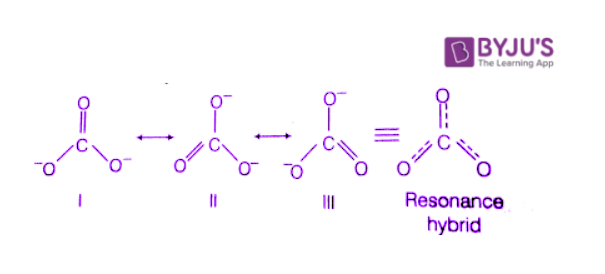15. Predict the hybridization of each carbon in the molecule of organic compound given below. Also indicate the total number of sigma and pi bonds in this molecule.

There are a total of 5 carbon atoms in the given structure:

• 2are sp hybridised and linked through a triple bond.
• 2are sp2 hybridised and linked through double bonds to O atoms.
• 1is sp3 hybridised and linked to two carbon atoms and two H atoms through single bonds.

There are 11σ bonds and 4 π bonds in the molecule.

16. Group the following as linear and non-linear molecules:
H2O, HOCI, BeCl2, Cl2O

Only BeCl2 is linear because the central atom O is surrounded by two lone pairs in the others.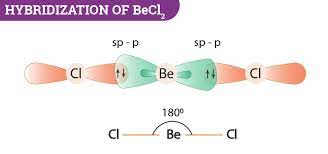Molecules are non-linear due to lone pair – lone pair repulsion.

H2O, HOCI, Cl2O are non-linear molecules.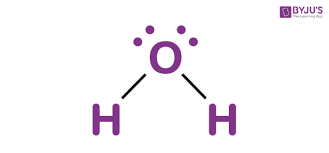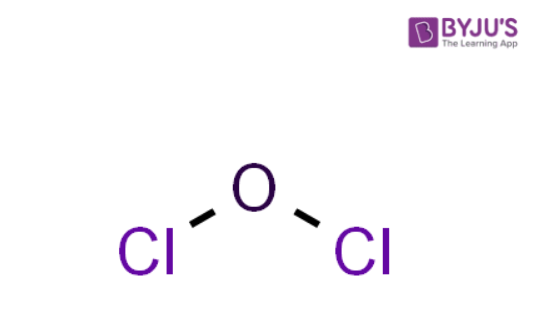17. Elements X, Y and Z have 4, 5 and 7 valence electrons respectively.

(i) Write the molecular formula of the compounds formed by these elements individually with hydrogen.

(ii) Which of these compounds will have the highest dipole moment?

(i) The molecular formula of the compounds are as follows:

• XH4
• YH3
• ZH1

(ii) X,Y, and Z each have 4,5 and 7 electrons. These elements are from the second period and the 14th, 15th, and 17th groups. The electronegativity of elements increases from group 1 to group 17.

As a result, H – Z will have the greatest dipole moment.

18. Draw the resonating structure of

(i) Ozone molecule

(ii) Nitrate ion

(i) Ozone molecule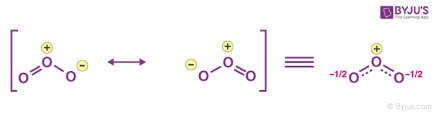(ii) Nitrate ion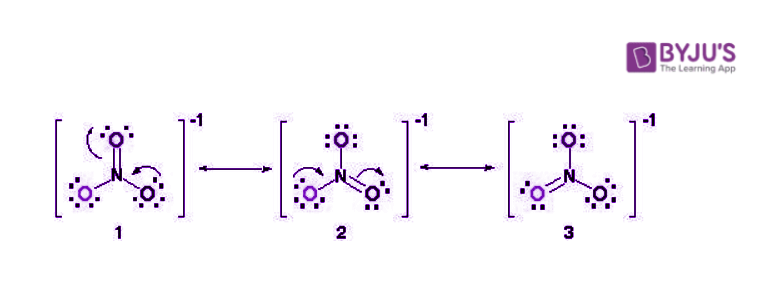19. Predict the shapes of the following molecules on the basis of hybridization.

BCI3, CH4, CO2, NH3

• BCl3 has sp2 hybridization and trigonal planar structure.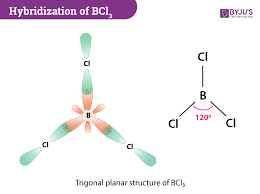• CH4 has sp3 hybridization and tetrahedral structure.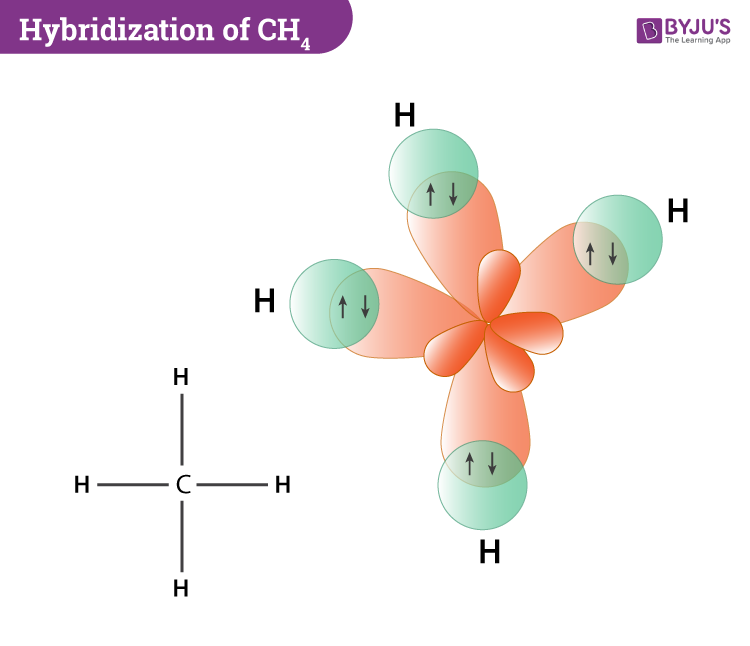• CO2 has sp hybridization and linear structure.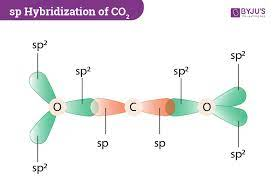• NH3 has sp hybridization and pyramidal structure.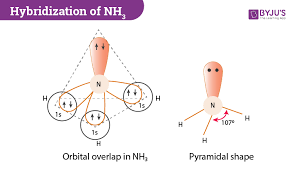20. All the C-O bonds in carbonate ion (CO32–) are equal in length. Explain.

Carbon is bonded to three oxygen atoms in carbonate ion. It has double bonds with two oxygen atoms and a single bond with one oxygen. Since bonds are not fixed and show resonance, all C – O bonds are the same length.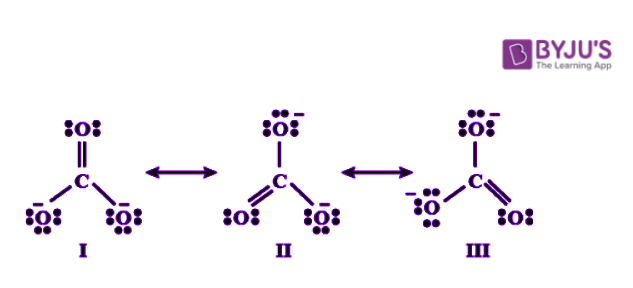21. What is meant by the term average bond enthalpy? Why is there a difference in bond enthalpy of O – H bond in ethanol (C2H-OH) and water?

Average Bond Enthalpy is calculated by dividing total bond dissociation enthalpy by the number of bonds broken.

All identical bonds in a molecule do not have the same bond enthalpies, for example, in water (H2O), there are two O – H bonds, but when the first O – H bond breaks, the second O – H bond changes due to charge. As a result, average bond enthalpy is used in polyatomic molecules and is calculated by dividing total bond dissociation enthalpy by the number of bonds broken.

H2O → H + OH; Δa H1 = 502 KJ/mol

OH → H + O; Δb H2 = 427 KJ/mol

Average Bond Enthalpy = (502 + 427)/2 = 464.5 KJ/mol.

Due to the different electronic environment around the oxygen atom, the bond enthalpies of O – H in C2H5OH and H2O differ.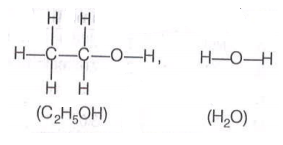In ethanol, O – H is attached to the carbon atom, whereas in water, O – H is attached to the hydrogen atom.

### Matching Type Questions

1. Match the species in Column I with the type of hybrid orbitals in Column II.

 Column I Column II (i) SF4 (a) sp3 d2 (ii) IF5 (b) d2 sp3 (iii) NO2+ (c) sp3d (iv) NH4+ (d) sp3 (e) sp

 Column I Column II (i) SF4 (c) sp3d (ii) IF5 (a) sp3 d (iii) NO2+ (e) sp (iv) NH4+ (d) sp3

2. Match the species in Column I with the geometry/shape in Column II.

 Column I Column II (i) H3O+ (a) Linear (ii) HC ≡ CH (b) Angular (iii) ClO2– (c) Tetrahedral (iv) NH4+ (d) Trigonal bipyramidal (e) Pyramidal

 Column I Column II (i) H3O+ (e) Pyramidal (ii) HC ≡ CH (a) Linear (iii) ClO2– (b) Angular (iv) NH4+ (c) Tetrahedral

3. Match the species in Column I with the bond order in Column II.

 Column I Column II (i) NO (a) 1.5 (ii) CO (b) 2.0 (iii) O2– (c) 2.5 (iv) O2 (d) 3.0

 Column I Column II (i) NO (c) 2.5 (ii) CO (d) 3.0 (iii) O2– (a) 1.5 (iv) O2 (b) 2.0

4. Match the items given in Column I with examples given in Column II.

 Column I Column II (i) Hydrogen bond (a) C (ii) Resonance (b) LiF (iii) Ionic Solid (c) H2 (iv)Covalent Solid (d) HF (e) O3

 Column I Column II (i) Hydrogen bond (d) HF (ii) Resonance (e) O3 (iii) Ionic Solid (b) LiF (iv)Covalent Solid (a) C

5. Match the shape of molecules in Column I with the type of hybridization in Column II.

 Column I Column II (i) Tetrahedral (a) sp2 (ii) Trigonal (b) sp (iii) Linear (c) sp3

 Column I Column II (i) Tetrahedral (c) sp3 (ii) Trigonal (a) sp2 (iii) Linear (b) sp

### Assertion and Reason Type Questions

In the following questions a statement of Assertion (A) followed by a statement of Reason (R) is given. Choose the correct option out of the choices given below each question.

1. Assertion (A): Sodium chloride formed by the action of chlorine gas on sodium metal is a stable compound.

Reason (R): This is because sodium and chloride ions acquire octet in sodium chloride formation.

(i) A and R both are correct, and R is the correct explanation of A.

(ii) A and R both are correct, but R is not the correct explanation of A.

(iii) A is true but R is false.

(iv) A and R both are false.

The correct option is (i) A and R both are correct, and R is the correct explanation of A.

Na (2, 8, 1) + Cl (2, 8, 7) → NaCl (2, 8)(2, 8, 8)

Sodium and chloride ions have complete octets in NaCl.

As a result, NaCl is a stable compound.

2. Assertion (A): Though the central atom of both NH3 and H2O molecules are sp3 hybridised, yet H-N-H bond angle is greater than that of H-O-H.

Reason (R): This is because nitrogen atom has one lone pair and oxygen atom has two lone pairs.

(i) A and R both are correct, and R is the correct explanation of A.

(ii) A and R both are correct, but R is not the correct explanation of A.

(iii) A is true but R is false.

(iv) A and R both are false.

The correct option is (i) A and R both are correct, and R is the correct explanation of A.

Lone pair has more repulsion than bond pair. Since H2O has 2 lone pairs, it has a smaller angle.

3. Assertion (A): Among the two O – H bonds in H20 molecule, the energy required to break the first O-H bond and the other O – H bond is the same.

Reason (R): This is because the electronic environment around oxygen is the same even after breakage of one O – H bond.

(i) A and R both are correct, and R is the correct explanation of A.

(ii) A and R both are correct, but R is not the correct explanation of A.

(iii) A is true but R is false.

(iv) A and R both are false.

The correct option is (iv) A and R both are false.

The enthalpy of the bond in H – O – H is not the same for both O – H bonds.

This is because the electronic charge on the oxygen atom changes after breaking one O – H bond

1. i) Discuss the significance/ applications of dipole moment.

(ii) Represent diagrammatically the bond moments and the resultant dipole moment in CO2, NF3 and CHCI3.

Dipole moment (μ) = charge (Q) ✕ separation distance (r). Debye units are commonly used to express dipole moment (D).

(a) It aids in the prediction of the polar and non-polar nature of compounds. Non-polar molecules have no dipole moment, whereas polar molecules have a dipole moment.

(b) It is possible to predict the nature of a chemical bond formed by knowing the electronegativities of the atoms involved in a molecule. The bond will be highly polar if the difference in electronegativities between two atoms is large. An ionic bond is formed when an electron is completely transferred from one atom to another.

The following formula can be used to calculate the percentage of ionic character:

(c) It is beneficial to understand the symmetry of the molecule. Despite having two or more polar bonds, symmetrical molecules have zero dipole moment.

In the case of BeF2, for example, the dipole moment is zero. This is due to the fact that the two equal bond dipoles point in opposite directions and cancel each other out.

(d) Differentiate between cis- and trans-isomers. Dipole moment measurements aid in the differentiation of cis- and trans-isomers because the ds-isomer has a higher dipole moment than the trans isomer.

(e) Distinguish between ortho, meta and para isomers. Dipole moment measurements aid in the differentiation of o-, m-, and p-isomers because the dipole moment of the p-isomer is zero and that of the o-isomers is greater than that of the m-isomer.

(ii) The bond moments and the resultant dipole moment in CO2, NF3 and CHCI3.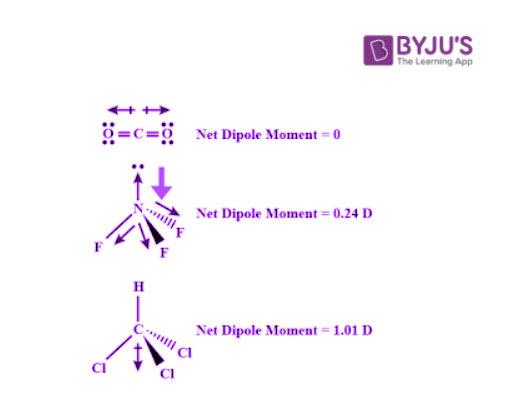2. Use the molecular orbital energy level diagram to show that N2 would be expected to have a triple bond, F2, a single bond and Ne2, no bond.

Formation of N2 molecule:

Electronic configuration is- σ1s2, σ∗1s2, σ2s2, σ∗2s2, π2px2 = π2py2, σ2pz2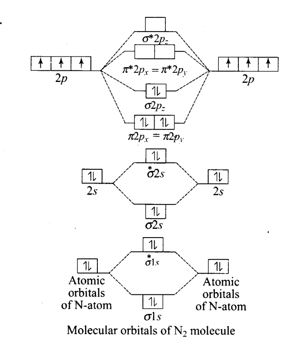Here, Nb = 10, Na = 4
Hence, Bond Order = ½ (Nb – Na) = ½ (10 – 4) = 3
Bond order indicates the number of bonds in diatomic molecule is 3. Hence, the molecule has TripleBond.

Formation of F2 molecule:

Electronic configuration is- σ1s2, σ∗1s2, σ2s2, σ∗2s2, σ2pz2, π2px2 = π2py2, π*2px2 = π*2py2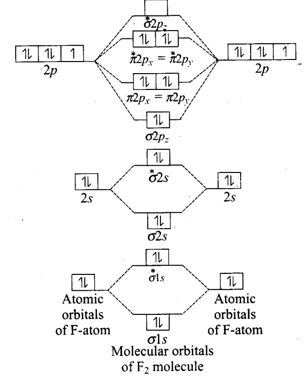Here, Nb = 10, Na = 8
Hence, Bond Order = ½ (Nb – Na) = ½ (10 – 8) = 1
Bond order indicates the number of bonds in diatomic molecule is 1. Hence, the molecule has a Single Bond.

Formation of Ne2 molecule:

Electronic configuration is- σ1s2, σ∗1s2, σ2s2, σ∗2s2, σ2pz2, π2px2 = π2py2, π*2px2 = π*2py2, σ*2pz2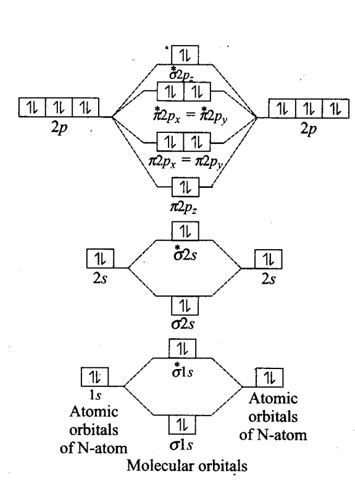Here, Nb = 10, Na = 10
Hence, Bond Order = ½ (Nb – Na) = ½ (10 – 10) = 0
Bond order indicates the number of bonds in diatomic molecule is 0. Hence, the molecule has no bond.

3. Briefly describe the valence bond theory of covalent bond formation by taking an example of hydrogen. How can you interpret energy changes taking place in the formation of dihydrogen?

Heitler and London proposed the valence bond theory in 1927. Later, in 1931, L. Pauling and J.C. Slater improved and developed it. The valence bond theory is based on knowledge of atomic orbitals and electronic configurations of elements, atomic orbital overlap criteria, and molecule stability.

The key concepts of valence bond theory are as follows:

(i) Atoms retain their identity even after the molecule is formed.

(ii) As the two atoms approach each other, the bond is formed by the interaction of only the valence electrons. The inner electrons are not involved in the formation of the bond.

(iii) Only the valence electrons of each bonded atom lose their identity during bond formation remain unaffected.

(iv) Bond stability is explained by the fact that bond formation is accompanied by the release of energy. At a certain distance between the atoms, the molecule has the least amount of energy. This is known as intemuclear distance. The stronger the bond formed, the greater the decrease in energy.

Consider the following two hydrogen atoms: A and B. Assume they are approaching each other, and their nuclei are NA and NB, respectively, and their electrons are eA and eB.

Assume that the two atoms are at a great distance from each other and that there is no interaction between them. They are now thought to be approaching each other, and new attractive and repulsive forces begin to operate.

We now understand that attractive forces exist between:

• Nucleus of one atom and its own electron, i.e., NA – eA and NB – eB.
• Nucleus of one atom and electron of another atom NA – eB and NB – eA.

Similarly, repulsive forces emerge between

• two atoms’ electrons eA – eB
• two atoms’ nuclei NA – NB

It has been discovered that the magnitude of the new attractive force is greater than the magnitude of the new repulsive forces. As a result, two atoms approach each other, reducing potential energy. Eventually, the net force of attraction equals the net force of repulsion, and the system acquires the least amount of energy. Two hydrogen atoms are said to be bonded together at this point to form a stable molecule with a bond length of 74pm.

Since the energy is released when the bond between two hydrogen atoms is formed, the hydrogen molecule is more stable than isolated hydrogen atoms. The energy released in this way is known as the bond enthalpy, and it corresponds to the minimum in the figure’s curve. In contrast, it takes 435.8kJ of energy to dissociate one mole of H2 molecule.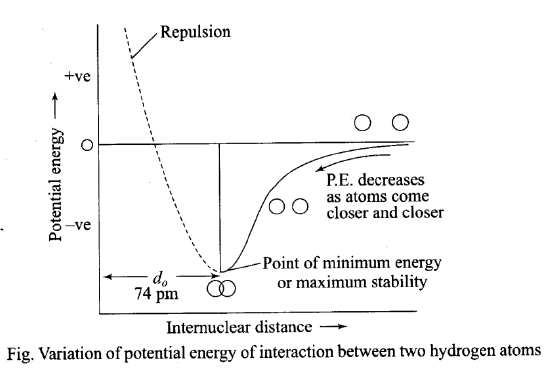Potential energy curve for the formation of an H2 molecule as a function of H atom internuclear distance. The curve’s minimum corresponds to the most stable state of H2.

4. Describe hybridization in the case of PCl5 and SF6. The axial bonds are longer as compared to equatorial bonds in PCl5 whereas in SF6, both axial bonds and equatorial bonds have the same bond length. Explain.

The ground state electronic configuration of P (15)- 1s2 2s2 3s2 3p3 3d0

The excited state outer electronic configuration- 1s2 2s2 3s1 3p3 3d1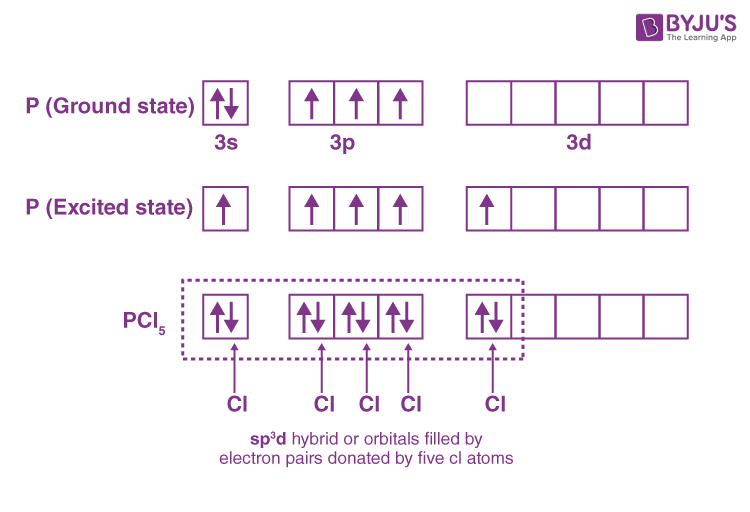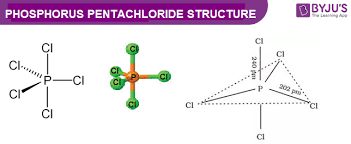Three P – Cl bonds are equatorial bonds because they are in the same plane and form a 120° angle with each other. The remaining two P – Cl bonds, one above and one below the equatorial plane, form a 90° angle with the plane. These are known as axial bonds. Because axial bond pairs experience more repulsive interaction than equatorial bond pairs, axial bonds have been found to be slightly longer and thus slightly weaker than equatorial bonds, making PCl5 molecules more reactive.

Since SF6 has (sp3d2) hybridization and an octahedral shape with bonds at 90° angles, all of the bonds in SF6 have the same bond length. As a result, the repulsion between all of the bonds is equal.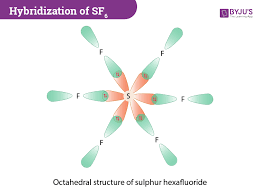5. (i) Discuss the concept of hybridization. What are its different types in a carbon atom?

(ii) What is the type of hybridization of carbon atoms marked with stars?

(i) The term “hybridization” was introduced by Pauling. The atomic orbitals, according to him, combine to form a new set of equivalent orbitals known as hybrid orbitals. In contrast to pure orbitals, hybrid orbitals are used in bond formation. The phenomenon is known as hybridization, which is defined as the process of intermixing orbitals with slightly different energies in order to redistribute their energies, resulting in the formation of a new set of orbitals with equivalent energies and shape.

For example, when one 2s and three 2p-orbitals of carbon hybridise, four new sp3 hybrid orbitals are formed.

The following are the main characteristics of hybridization:

1. The number of hybrid orbitals equals the number of atomic orbitals hybridised.
2. The hybridised orbitals are always energy and shape equivalent.
3. Hybrid orbitals are more effective than pure atomic orbitals at forming stable bonds.
4. These hybrid orbitals are oriented in space in a preferred direction to achieve the least amount of repulsion between electron pairs and thus a stable arrangement.

As a result, the type of hybridization indicates the molecule’s geometry.

• In carbon compounds, if carbon is linked to carbon via a triple bond, such as alkynes, triple bonded carbon is sp hybridised.
• In carbon compounds, if carbon is linked to carbon via a double bond (C=C), such as in alkenes, the double-bonded carbon is sp2 hybridised.
• In carbon compounds, if carbon is linked to carbon via a single bond (C – C), such as alkanes, the carbon is sp3 hybridised.

(ii) Type of hybridization in the figure:

1. Both atoms have sp2 hybridization.
2. The marked atom is sp3 hybridised.
3. The marked atom is sp2 hybridised.
4. The marked atom is sp3 hybridised.
5. The marked atom is sp hybridised.

### Comprehension given below is followed by some multiple choice questions. Each question has one correct option. Choose the correct option.

Molecular orbitals are formed by the overlap of atomic orbitals. Two atomic orbitals combine to form two molecular orbitals called bonding molecular orbital (BMO) and antibonding molecular orbital (ABMO). Energy of antibonding orbital is raised above the parent atomic orbitals that have combined and the energy of the bonding orbital is lowered than the parent atomic orbitals. Energies of various molecular orbitals for elements hydrogen to nitrogen increase in the order :

σ1s < σ*1s < 1σ2s < σ*2s <(π2px≈π2py > <σ2p₂<(π*2px-π*2p,)<σ*2p, and for oxygen and fluorine order of energy of molecular orbitals is given below: σ1s < σ*1s < σ2sσ*2s < σp,(π2px≈π2py) <(π*2px≈π2py)<σ*2pz

Different atomic orbitals of one atom combine with those atomic orbitals of the second atom which have comparable energies and proper orientation. Further, if the overlapping is head on, the molecular orbital is called ‘Sigma’, (σ) and if the overlap is lateral, the molecular orbital is called ‘pi’, (π). The molecular orbitals are filled with electrons according to the same rules as followed for filling of atomic orbitals. However, the order for filling is not the same for all molecules or their ions. Bond order is one of the most important parameters to compare the strength of bonds.

6. Which of the following statements is correct?

(i) In the formation of dioxygen from oxygen atoms 10 molecular orbitals will be formed.

(ii) All the molecular orbitals in the dioxygen will be completely filled.

(iii) Total number of bonding molecular orbitals will not be same as total number of antibonding orbitals in dioxygen.

(iv) Number of filled bonding orbitals will be same as number of filled antibonding orbitals.

The correct answer is (i) In the formation of dioxygen from oxygen atoms 10 molecular orbitals will be formed.

Oxygen has atomic configuration 1s2, 2s2, 2px2 2py1 2pz1, which means 5 orbitals. Two oxygen atoms overlap five atomic orbitals each and thus, forming ten molecular orbitals.

7. Which of the following molecular orbitals has maximum number of nodal planes?

(i) σ∗1s

(ii) σ∗2px

(iii) π2px

(iv) π∗2py

The correct answer is (iv) π∗2py

8. Which of the following pair is expected to have the same bond order?

(i) O2, N2

(ii) O2+, N2

(iii) O2, N2+

(iv) O2, N2

The correct answer is (ii) O2+, N2

9. In which of the following molecules, σ2pz molecular orbital is filled after π2px and π2py molecular orbitals?

(i) O2

(ii) F2

(iii) N2

(iv) None of these

The correct answer is (iii) N2.

Test your knowledge on Important Questions Class 11 Chemistry Chapter 4 Chemical Bonding Molecular Structure